### 树脂复合圆形井盖

#### 作者：江苏常州巨龙建筑材料厂 来源：本站 发布日期：2021-07-06 14:40:58 点击次数：2494

Φ300*25=18

Φ400*20=25

Φ400*40=35

Φ500*35=58

Φ500*50=80

Φ600*35=62

Φ600*50=100

Φ700*30=58

Φ700*40=88

Φ700*50=128

Φ700*70=188

Φ800*40=125

Φ800*50=200

Φ800*70=288

Φ900*60=260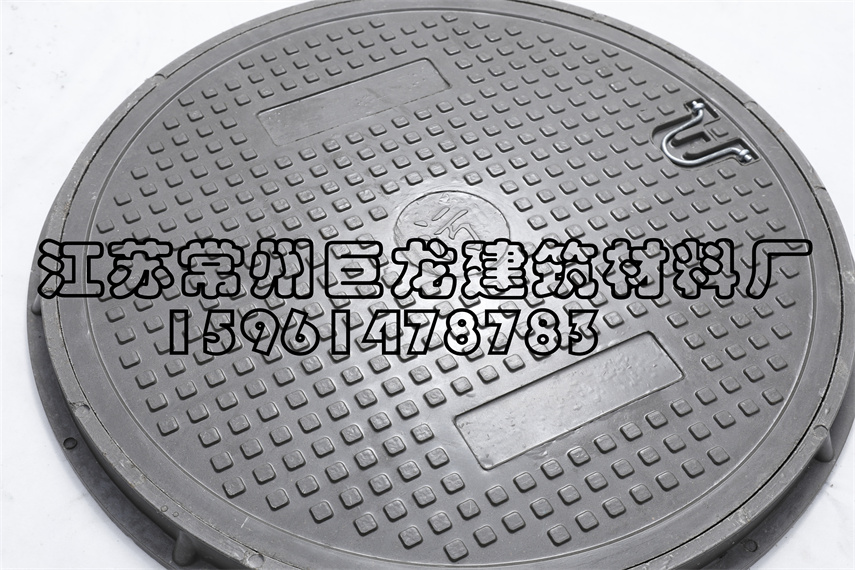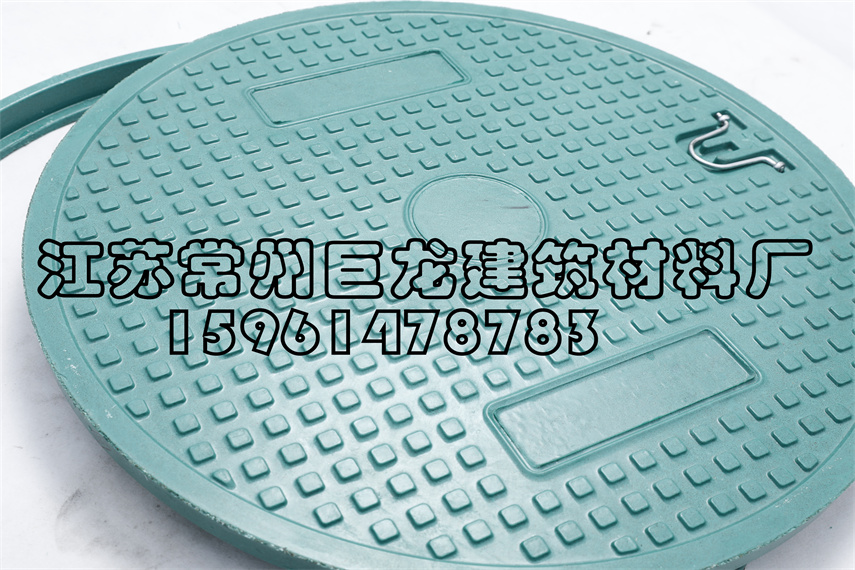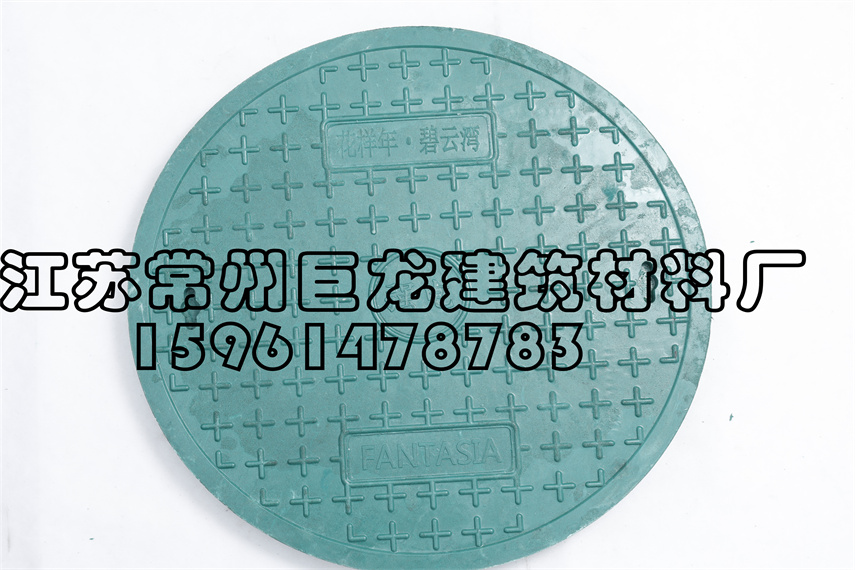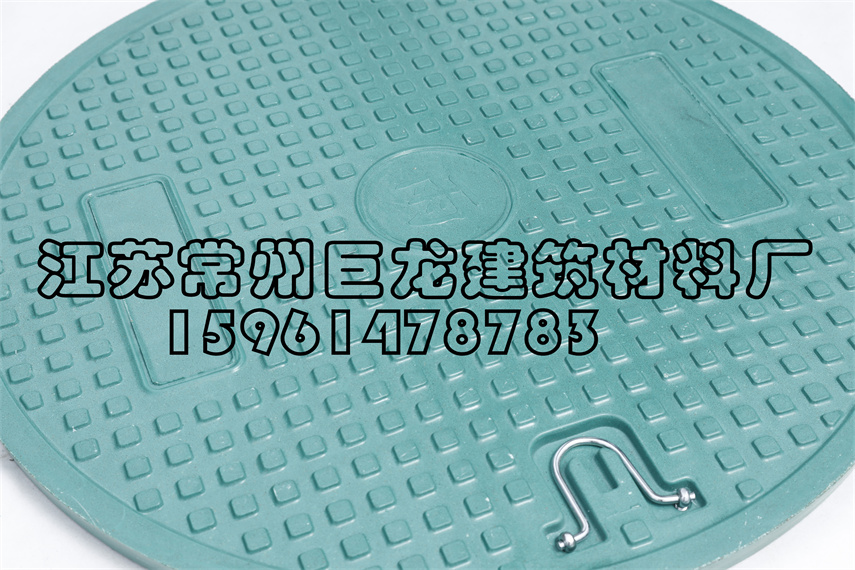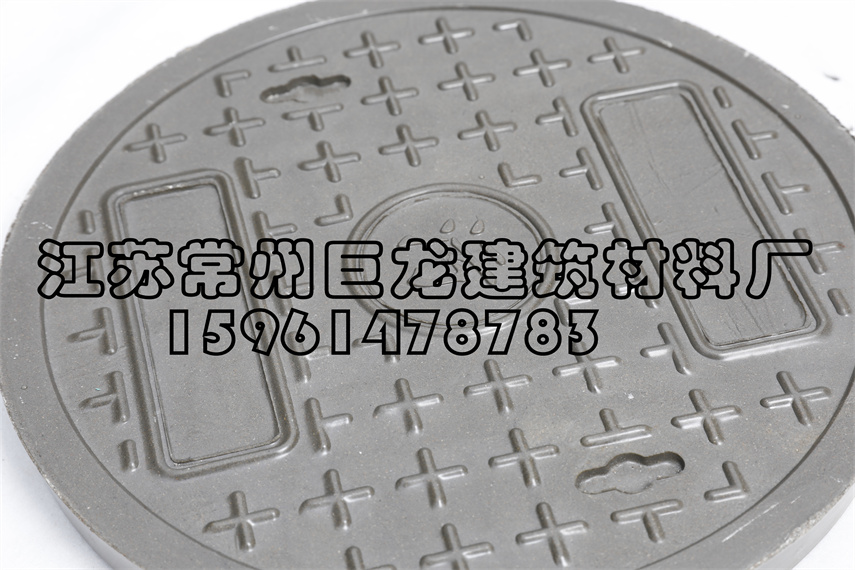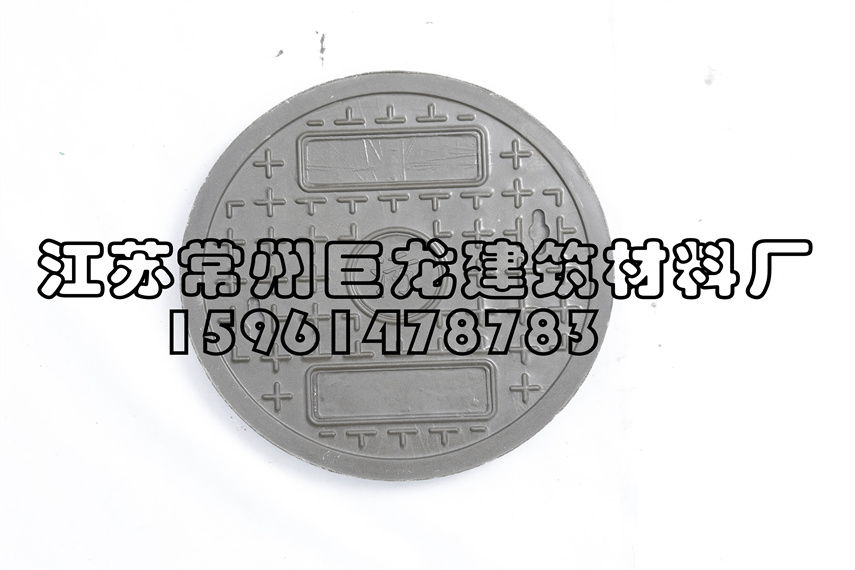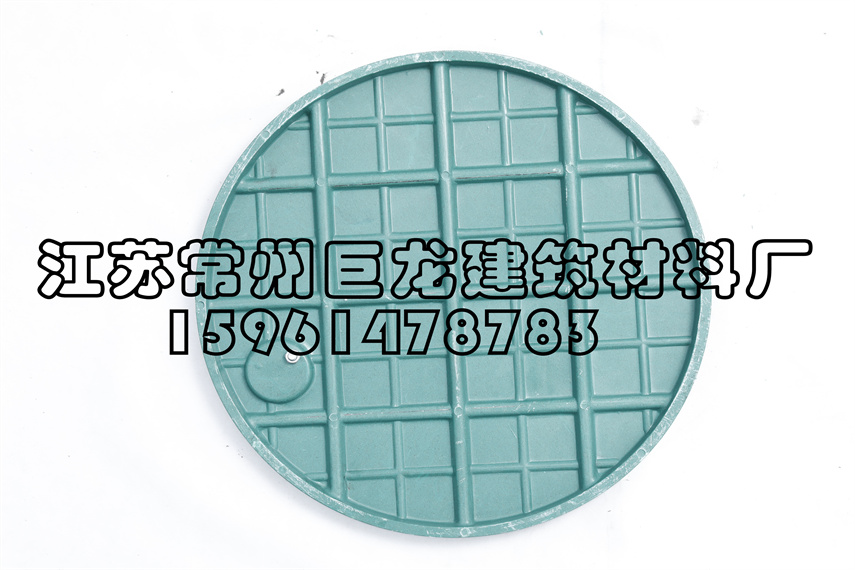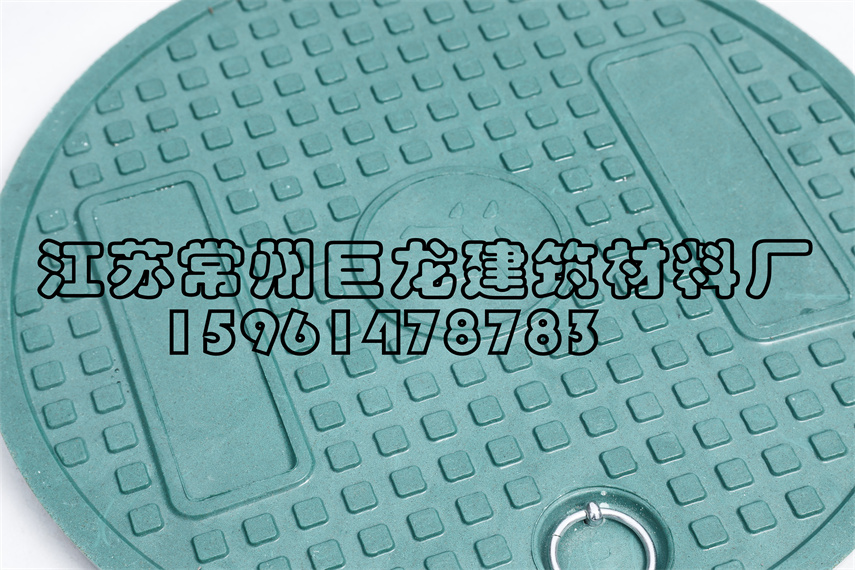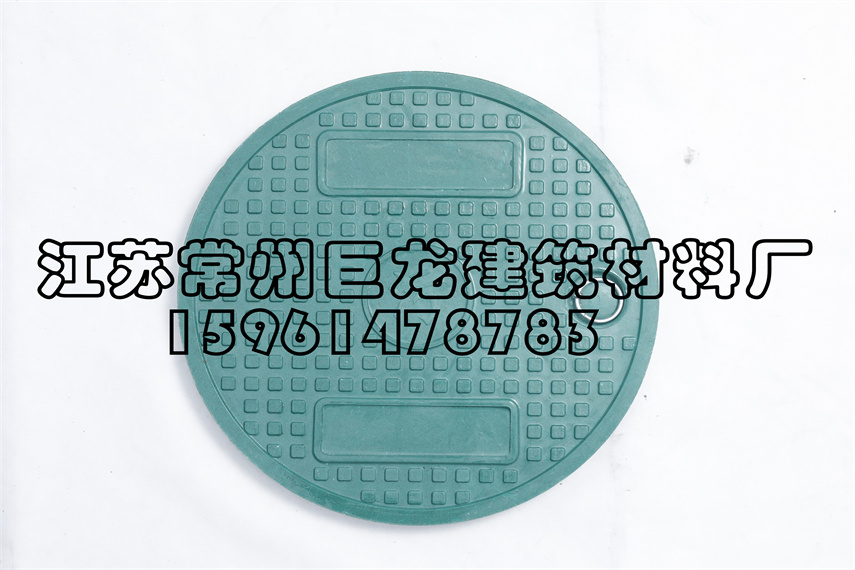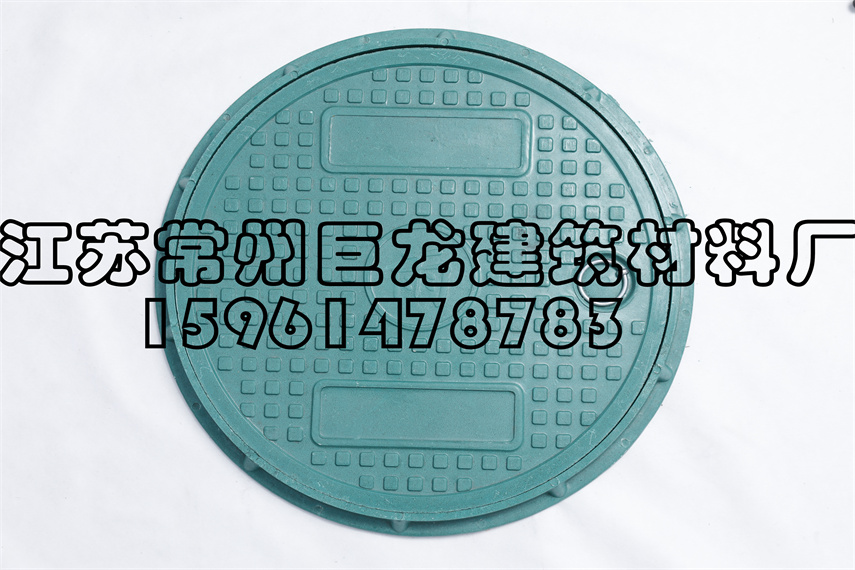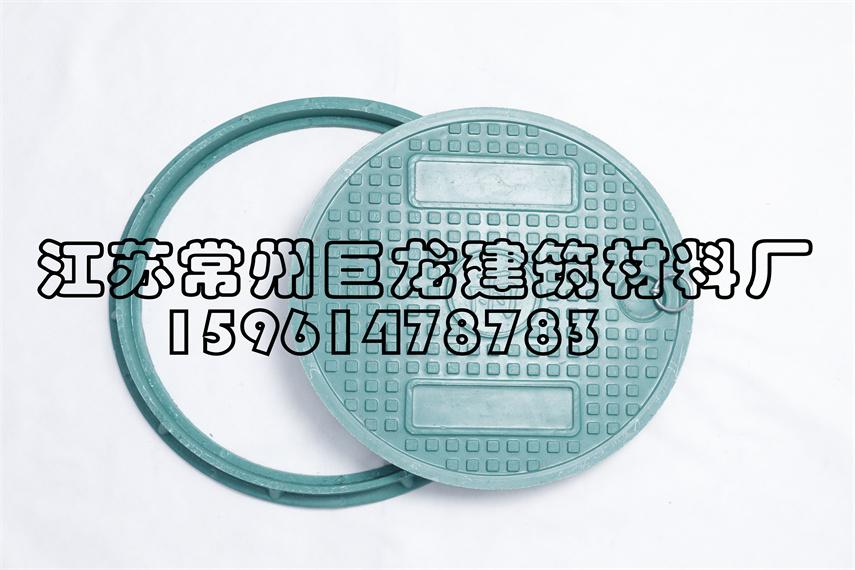公司名称： 联系人： 联系电话： 联系邮箱： 联系地址： 备注：
##### 联系我们

 姓名： 电话： 留言：

• 15961478783
•• 点击扫描下载